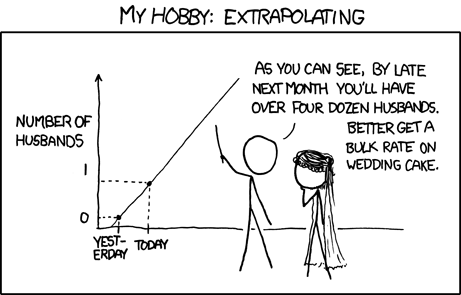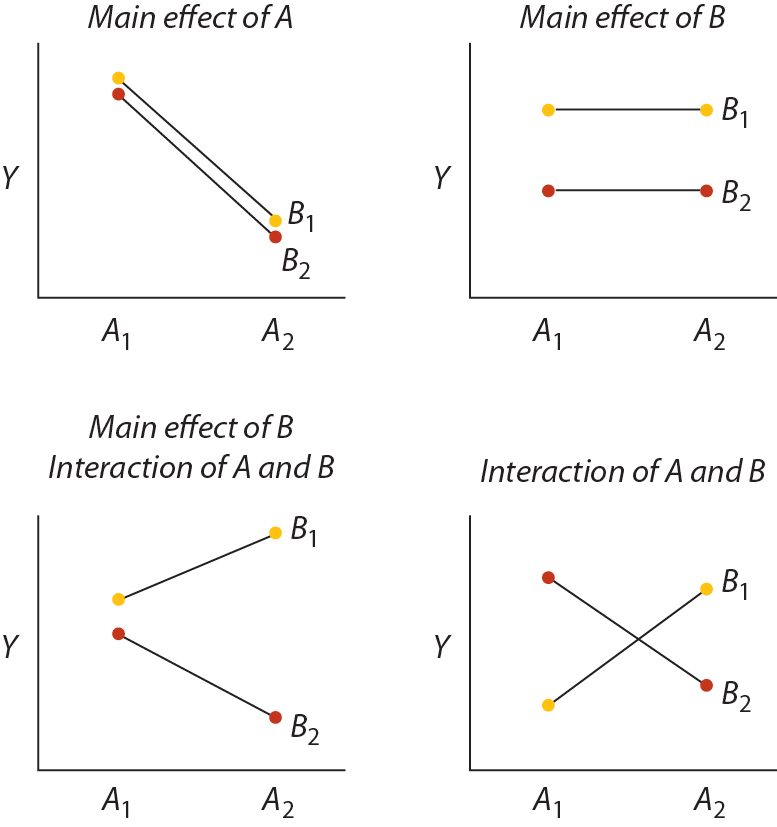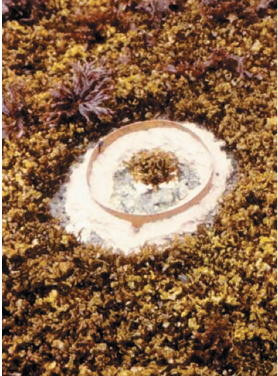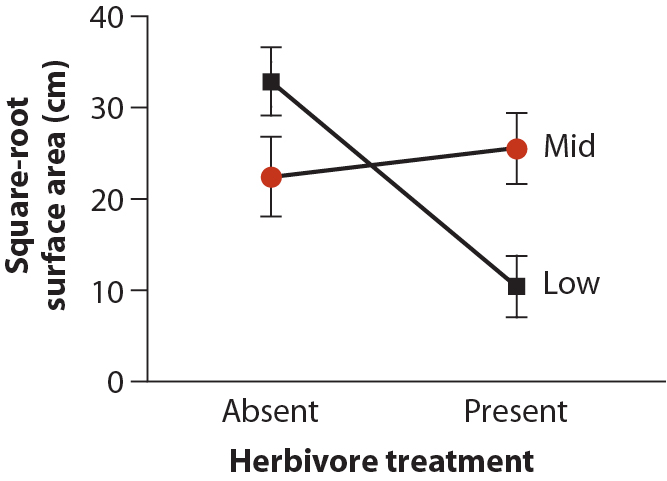Multiple explanatory variables (cont'd)

M. Drew LaMar
April 27, 2016https://xkcd.com/605/

Factorial designs using GLMs

Linear model Other name Example study design
$$Y = \mu + A + B + A*B$$ Two-way, fixed-
effects ANOVA
Factorial experiment
$$Y = \mu + A + b + A*b$$ Two-way, mixed-
effects ANOVA
Factorial experiment
$$Y = \mu + X + A$$ Analysis of covariance (ANCOVA) Observational study

The explanatory variables are called factors, as they represent treatments of direct interest.

$$\mathrm{A}$$ and $$\mathrm{B}$$ are called main effects; they represent effects of each factor alone, when averaged over the categories of the other factor.

Factorial designs using GLMs

Linear model Other name Example study design
$$Y = \mu + A + B + A*B$$ Two-way, fixed-
effects ANOVA
Factorial experiment
$$Y = \mu + A + b + A*b$$ Two-way, mixed-
effects ANOVA
Factorial experiment
$$Y = \mu + X + A$$ Analysis of covariance (ANCOVA) Observational study

The explanatory variables are called factors, as they represent treatments of direct interest.

$$\mathrm{A*B}$$ is the interaction term.

Factorial designs using GLMsFactorial designs using GLMs

Example 18.3: Interaction zoneFactorial designs using GLMsHarley (2003) investigated how herbivores affect the abundance of plants living in the intertidal habitat of coastal Washington using field transplants of a red alga, Mazzaella parksii. The experiment also examined whether the effect of herbivores on the algae depended on where in the intertidal zone the plants were growing. Thirty-two study plots were established just above the low-tide mark, and another 32 plots were set at mid-height between the low- and high-tide marks. Using copper fencing, herbivores were excluded from a randomly chosen half of the plots at each height.

Factorial designs using GLMs\begin{align} \mathrm{ALGAE} = & \mathrm{CONSTANT} + \mathrm{HERBIVORY} + \mathrm{HEIGHT} +\\ & \mathrm{HERBIVORY*HEIGHT} \end{align}Factorial designs using GLMs\begin{align} \mathrm{ALGAE} = & \mathrm{CONSTANT} + \mathrm{HERBIVORY} + \mathrm{HEIGHT} +\\ & \mathrm{HERBIVORY*HEIGHT} \end{align}

We need to examine the improvement in fit of the model to the data with and without each term (i.e. main effects and interaction effect).

Question: Does herbivory have an effect on mean algal cover?

Null Model (Type I): $\mathrm{ALGAE} = \mathrm{CONSTANT}$

Alt Model: $\mathrm{ALGAE} = \mathrm{CONSTANT} + \mathrm{HERBIVORY}$